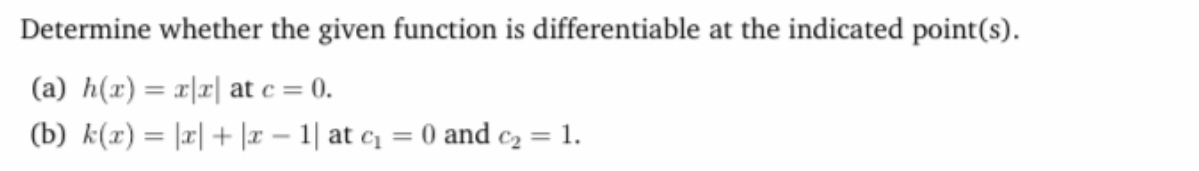# Determine whether the given function is differentiable at the indicated point(s). (a) h(x) = x|r| at c = 0. %3D (b) k(x) = |r| + |r – 1| at c = 0 and c2 = 1.

Questionhelp_outlineImage TranscriptioncloseDetermine whether the given function is differentiable at the indicated point(s). (a) h(x) = x|r| at c = 0. %3D (b) k(x) = |r| + |r – 1| at c = 0 and c2 = 1. fullscreen

### Want to see this answer and more?

Experts are waiting 24/7 to provide step-by-step solutions in as fast as 30 minutes!*

*Response times may vary by subject and question complexity. Median response time is 34 minutes for paid subscribers and may be longer for promotional offers.
Tagged in
Math